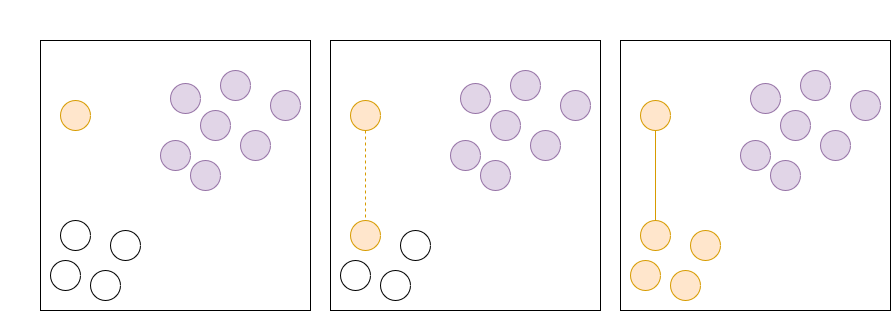While reading Judson’s Abstract Algebra book (described more in this post) I also learned about equivalence relations. This topic is covered early in the book as part of a review about sets.

I think equivalence relations represent a very elegant idea. If you can create an “equivalence” between two objects in a set then you can use the same equivalence relation to partition the set into distinct non-overlapping subsets.

## Definition#

An equivalence relation is an operation on two elements that returns true or false. For example, $x \sim y$ is true if $x$ is equivalent to $y$.

There are three requirements for this operation to be an equivalence relation:

• Reflexive: $x \sim x$ for all $x$. This means that x is always equivalent to itself.
• Symmetric: $x \sim y$ means $y\sim x$.
• Transitive: $x \sim y$ and $y \sim z$ means $x \sim z$

Note that by this definition “approximation” are not an equivalence relation. For example, we may have that $1.01 \approx 1.02$ and $1.02 \approx 1.03$ and so on to $1.08 \approx 1.09$ but we probably also want that $1.01 \not \approx 1.09$. This violates our transitive condition.

## Partition#

The transitive condition is what gives the equivalence relation its power. The transitive condition means that the equivalence condition is “infective”.Diagram of an equivalence relation ‘infecting’ a group of objects

We start with three different groups that are equivalent within. In the second image, it is found that the orange object is equivalent to one of the white objects. Because of the transitive property, this means that all the white objects are equivalent to the orange object.

This is why an equivalence relation allows you to divide a set into distinct non-overlapping subsets known as partitions or equivalence classes.

## Examples#

### Trivial Partitions#

All sets have two trivial equivalence relations:

• Each element is only equivalent to itself. This results in a partition where each element is in its own subset.
• All elements are equivalent to each other. This results in the whole set being the “partition”.

Both of these partitions are somewhat useless since they don’t give us any more information about the set. However, it highlights how a “useful” equivalence relation has the right level of connectedness.

### Boolean#

An incrementally more useful equivalence relation divides the set into two. Suppose you have a function $f$ that accepts an element of a set and returns true or false. For example, if the set is a list of cars, we can define $f$ to be true when the car is red, otherwise it is false. Then we can define $x \sim y$ if $f(x) = f(y)$. This will create a partition of all elements where $f(x)$ returns true and another partition where $f(x)$ returns false.

Practically this is what we do when we query a system. When you load your email, the system queries for all emails that have been sent to you. “Emails for you” becomes the $f$ function and the system returns the partition for which $f$ is true. Similarly, we can think that databases do the same for us. We tell the database what $f$ is and it returns all the records that match the conditions for $f$. The database partitions all the record by $f$ and returns the partition where $f$ was true.

### Multivalued#

The next incremental step we can take is to change $f$ to return any value. For example, if the set is a list of cars, $f$ could return the color of the car. If the set is people, $f$ could return people’s height. If the set is a list of items in a store, then $f$ could return the price of each item.

In each case, the equivalence relation can be defined as $x \sim y$ if $f(x) = f(y)$.

This will result in partitions where $f$ has the same value in each partition. For cars, the partitions will be cars grouped by color. For people, the partition will be people grouped by height. Similarly, for store items, the partition will be items grouped by price.

### Crazier#

The examples above feel natural as you can see how the grouping action works. Judson gives a crazier example of similar matrices.

Suppose we have two square matrices A and B of size $2 \times 2$. Then $A \sim B$ if there exists a third matrix $C$ such that $C A C^{-1} = B$.

This relation is reflexive ($A \sim A$) because we can let $C$ be the identity matrix which would give us $IAI^{-1} = A$.

This relation is symmetric because we can change $C$ to $C^{-1}$ and have the same equality hold. The book provides the proof for how this relation is transitive but it boils down to matrix multiplication being associative.

Since the relation fulfills all the requirements of being an equivalence relation, we know that this relation partitions the set! Even though at a first glance it is hard to see how the relation creates non-overlapping subsets of matrices, we know they exist.

It turns out that a partition represents the subset of matrices that result in the same linear transformation under some bases.

## Conclusion#

The last example captures why I found the concept of equivalence relations appealing. Finding equivalences and partitioning a set feel like two very different problems. However, if you can solve one, you automatically get a solution for the other. That feels very powerful.